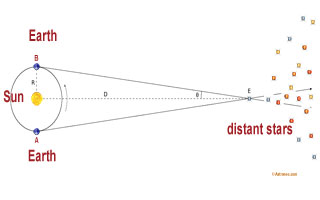# Astronomical unit (AU)

## Astronomical unit, new definitionAutomatic translation Category: Sun
Updated June 01, 2013

Among the units of measure astronomical distances, the astronomical unit (UA symbol) is the smallest units, it corresponds approximately to the length of the semi-major axis of Earth's orbit (the mean distance of the Earth Sun).
On 21 September 2012, the Paris Observatory issued a press release on the new definition of the astronomical unit is now fixed. At its General Assembly held in Beijing from 20 to 31 August 2012, the International Astronomical Union (IAU) adopted a new definition of the astronomical unit, unit of length used by astronomers to express the dimensions of the solar system. The unit of length is exactly 149 597 870 700 meters, conventional value chosen to be compatible with the system of astronomical constants in force since 2009. The astronomical unit is approximately equal to the Earth-Sun distance, was created in 1958 to measure the distances of objects in the solar system.
At the time, his purpose was to help give exact values ​​of relative distances in the solar system because it was not possible to estimate distances in meters with high precision as today.
"The definition that was in effect before August 30, 2012 based on a mathematical expression involving the mass of the Sun (MS), day length (D) and a constant k (constant Gauss) whose numerical value was fixed by convention. In addition, its value was determined experimentally meters so that it depended on models and observations used and the reference system chosen. This value was used to derive the SI (International System of Units) solar mass parameter, which was thus determined only indirectly. However, the dynamic contemporary astronomy requires to be placed in the context of general relativity and use a consistent set of units and constants. The definition of the astronomical unit therefore required revision. The astronomical unit is commonly used in astronomy, this resolution affects all sciences of the Universe.", Says the Paris Observatory.

However, this change mainly concerns the field of high-precision dynamic solar system.
Although the astronomical unit defines the parsec, the relative difference between the two definitions, old and new, does not exceed 0.000 000 01%.
As a reminder, a parsec (parallax second) is defined as the distance at which one astronomical unit subtends an angle of one second of arc.
This new definition will have no significant effect, given the relative errors of cosmic distances outside the solar system, however, astronomers now have a perfectly defined, consistent with general relativity and reports directly to the International System of Units (SI) through the meter.
We retain an AU is approximately 150 million km, the Earth-Sun distance.

 Planets Distances (AU) Mercury 0,38 Venus 0,72 Earth 1,00 Mars 1,52 Asteroid belt 2 - 3,5 Jupiter 5,21 Saturn 9,54 Uranus 19,18 Neptune 30,11 Kuiper Belt 30 à 55 Oort cloud 50 000 Light-year 63 242 Parsec 206 264,806### Image: The new definition of the astronomical unit 2012 (149,597,870,700 meters) will have no effect on distances outside the solar system, taking into account current approximations cosmic distances. However, astronomers have since 2012, a perfectly defined, consistent with general relativity and reports directly to the International System of Units (SI) through the meter.

nota: Three units of measure in astronomy useful for expressing distances.
- A
light year is 242.17881 63 AU is exactly equal to 9 460 895 288 762 850 meters.
- A
parsec is equal to 206 AU or 270.6904 3.2616 years is 30 light 857 656 073 828 900 meters.
- An
astronomical unit is from August 30, 2012, exactly 149 597 870 700 meters.

1997 © Astronoo.com − Astronomy, Astrophysics, Evolution and Earth science.The distance of stars...How to calculate the distance to the horizon?